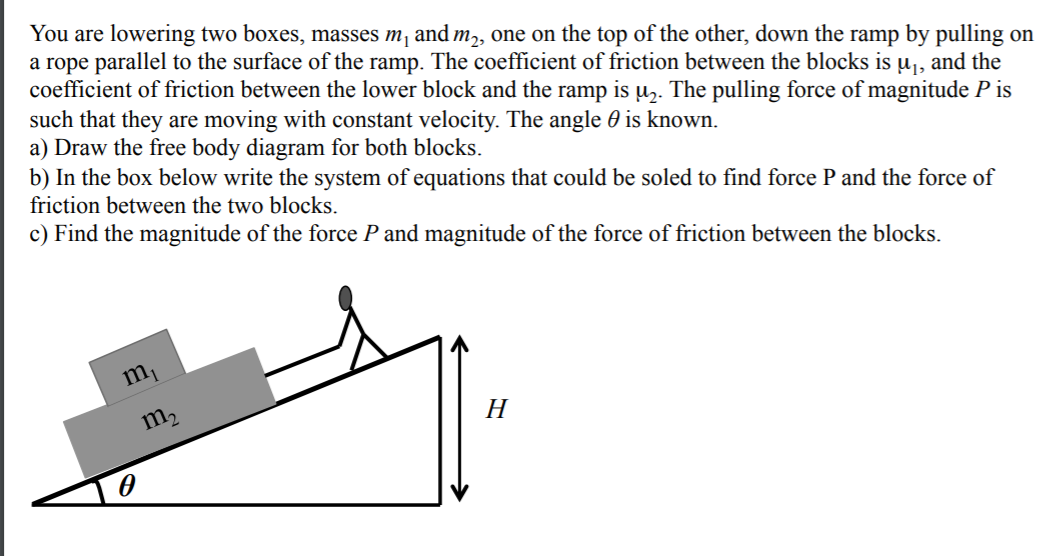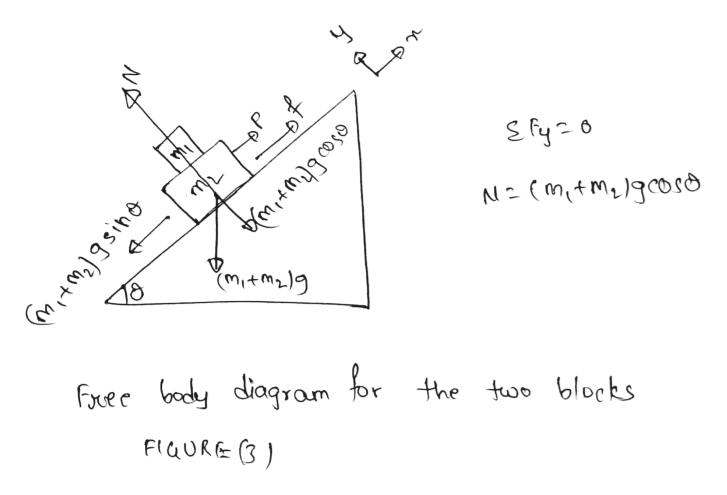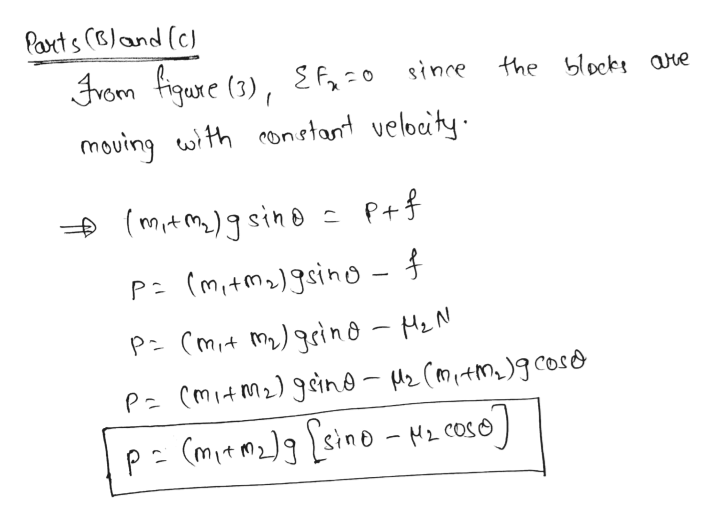# You are lowering two boxes, masses m, and m,, one on the top of the other, down the ramp by pulling ona rope parallel to the surface of the ramp. The coefficient of friction between the blocks is u̟, and thecoefficient of friction between the lower block and the ramp is uz. The pulling force of magnitude P issuch that they are moving with constant velocity. The angle 0 is known.a) Draw the free body diagram for both blocks.b) In the box below write the system of equations that could be soled to find force P and the force offriction between the two blocks.c) Find the magnitude of the force P and magnitude of the force of friction between the blocks.m,

Question
28 views

need help on pictured problemhelp_outlineImage TranscriptioncloseYou are lowering two boxes, masses m, and m,, one on the top of the other, down the ramp by pulling on a rope parallel to the surface of the ramp. The coefficient of friction between the blocks is u̟, and the coefficient of friction between the lower block and the ramp is uz. The pulling force of magnitude P is such that they are moving with constant velocity. The angle 0 is known. a) Draw the free body diagram for both blocks. b) In the box below write the system of equations that could be soled to find force P and the force of friction between the two blocks. c) Find the magnitude of the force P and magnitude of the force of friction between the blocks. m, fullscreen
check_circle

Step 1
Step 2help_outlineImage TranscriptioncloseÎo N=(mit Me)gcoso (mitmz)g 18 Free body diagram for the two blocks FIQURE (3) fullscreen
Step 3help_outlineImage TranscriptioncloseParts (Bland (c) From figure (3), E f, =0 sinre the blocks are mouing with constant velocity. + (m,tm)gsino = P+f P= (mitmz) gsino - { P- (mit my) grino - MzN P= (mitM2) gsino - Mz (Mitma)gcoso p= (mitm2]g [sino - Hz cOso) fullscreen

### Want to see the full answer?

See Solution

#### Want to see this answer and more?

Solutions are written by subject experts who are available 24/7. Questions are typically answered within 1 hour.*

See Solution
*Response times may vary by subject and question.
Tagged in

### Science